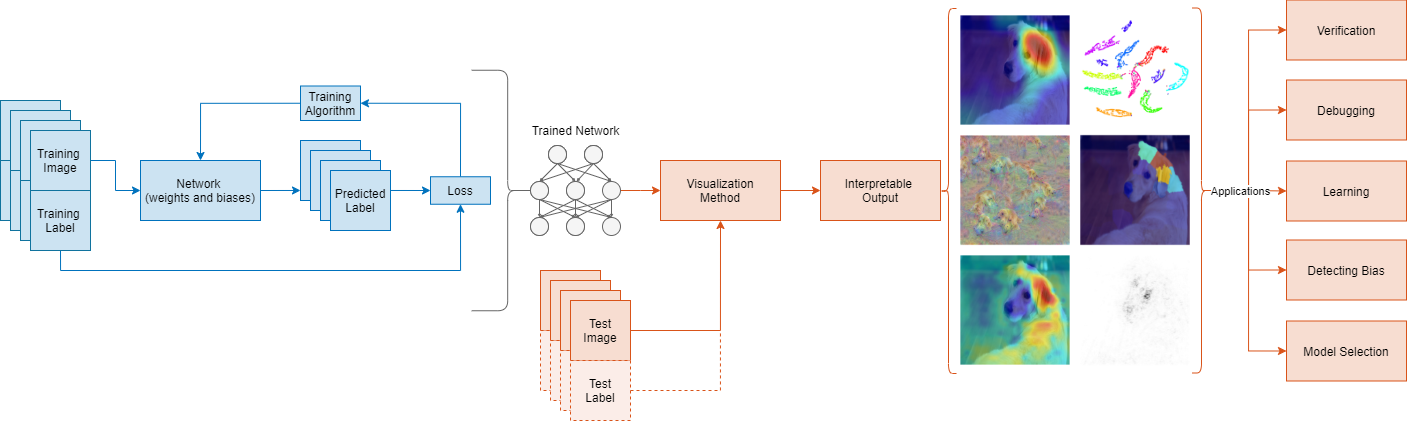# Visualization and Interpretability

Plot training progress, assess accuracy, explain predictions, and visualize features learned by a network

Monitor training progress using built-in plots of network accuracy and loss. Investigate trained networks using visualization techniques such as Grad-CAM, occlusion sensitivity, LIME, and deep dream.

Deep Learning Visualization MethodsDeep Learning Visualization Methods

## Apps

 Deep Network Designer Design, visualize, and train deep learning networks

## Objects

 `trainingProgressMonitor` Monitor and plot training progress for deep learning custom training loops (Since R2022b)

## Functions

expand all

 `analyzeNetwork` Analyze deep learning network architecture `plot` Plot neural network architecture
 `updateInfo` Update information values for custom training loops (Since R2022b) `recordMetrics` Record metric values for custom training loops (Since R2022b) `groupSubPlot` Group metrics in training plot (Since R2022b)
 `accuracyMetric` Deep learning accuracy metric (Since R2023b) `aucMetric` Deep learning area under ROC curve (AUC) metric (Since R2023b) `fScoreMetric` Deep learning F-score metric (Since R2023b) `precisionMetric` Deep learning precision metric (Since R2023b) `recallMetric` Deep learning recall metric (Since R2023b) `rmseMetric` Deep learning root mean squared error metric (Since R2023b)
 `activations` Compute deep learning network layer activations `confusionchart` Create confusion matrix chart for classification problem `sortClasses` Sort classes of confusion matrix chart `rocmetrics` Receiver operating characteristic (ROC) curve and performance metrics for binary and multiclass classifiers (Since R2022b) `addMetrics` Compute additional classification performance metrics (Since R2022b) `average` Compute performance metrics for average receiver operating characteristic (ROC) curve in multiclass problem (Since R2022b) `plot` Plot receiver operating characteristic (ROC) curves and other performance curves (Since R2022b)
 `imageLIME` Explain network predictions using LIME (Since R2020b) `occlusionSensitivity` Explain network predictions by occluding the inputs (Since R2019b) `deepDreamImage` Visualize network features using deep dream `gradCAM` Explain network predictions using Grad-CAM (Since R2021a)

## Properties

 ConfusionMatrixChart Properties Confusion matrix chart appearance and behavior ROCCurve Properties Receiver operating characteristic (ROC) curve appearance and behavior (Since R2022b)